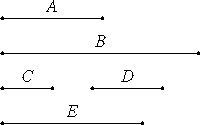# Proposition 14

If a square measures a square, then the side also measures the side; and, if the side measures the side, then the square also measures the square.

Let A and B be square numbers, let C and D be their sides, and let A measure B.

I say that C also measures D.as in VIII.11

Multiply C by D to make E. Then A, E, and B are continuously proportional in the ratio of C to D.

And, since A, E, and B are continuously proportional, and A measures B, therefore A also measures E. And A is to E as C is to D, therefore C measures D.

Next, let C measure D.

I say that A also measures B.

VII.Def.20

With the same construction, we can in a similar manner prove that A, E, and B are continuously proportional in the ratio of C to D. And since C is to D as A is to E, and C measures D, therefore A also measures E.

And A, E, and B are continuously proportional, therefore A also measures B.

Therefore, if a square measures a square, then the side also measures the side; and, if the side measures the side, then the square also measures the square.

Q.E.D.

## Guide

If c2 divides d2, then c divides d.

This proposition is used to prove its contrapositive, VIII.16.